# MATLAB scripts for alternating direction method of multipliers

S. Boyd, N. Parikh, E. Chu, B. Peleato, and J. Eckstein

This page gives MATLAB implementations of the examples in our paper on distributed optimization with the alternating direction method of multipliers.

These scripts are serial implementations of ADMM for various problems. In cases where the scripts solve distributed consensus problems (e.g., distributed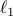-regularized logistic regression), the code runs serially instead of in parallel. These scripts give an idea of the structure and flavor of ADMM; an implementation in C/C++ that follows the structure laid out in our scripts and exploits parallelism can be competitive with state-of-the-art solvers for these problems. These are presented in MATLAB for readability. Unless otherwise noted on this page, most scripts stand alone and work in Matlab without further modification. Some scripts may require CVX.

As output, each example script displays the primal residual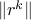, the primal feasibility tolerance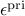, the dual residual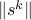, and the dual feasibility tolerance. See section 3.3 of the paper for more details on these quantities. Also included are plots of the objective value and the primal and dual residual by iteration. Note that the objective value at any particular iteration can go below the true solution value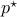because the iterates need not be feasible (e.g., if the constraint is, we can have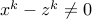for some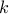).

You can use the grabcode function built in to MATLAB to obtain these codes. Please report any issues to the authors.

## Examples

1. Basis pursuit

2. Sparse inverse covariance selection

3. Huber fitting

4. Intersection of polyhedra

5. Lasso

6. Lasso with LSQR, an iterative method for solving sparse systems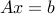7. Least absolute deviations

8. Linear programming

9.-regularized logistic regression

10. Distributed-regularized logistic regression

1. ADMM function - also requires l2_log, l2_log_grad, record_bfgs_iters, and LBFGS-B for Matlab.

2. Example

11. Regressor selection (nonconvex problem)